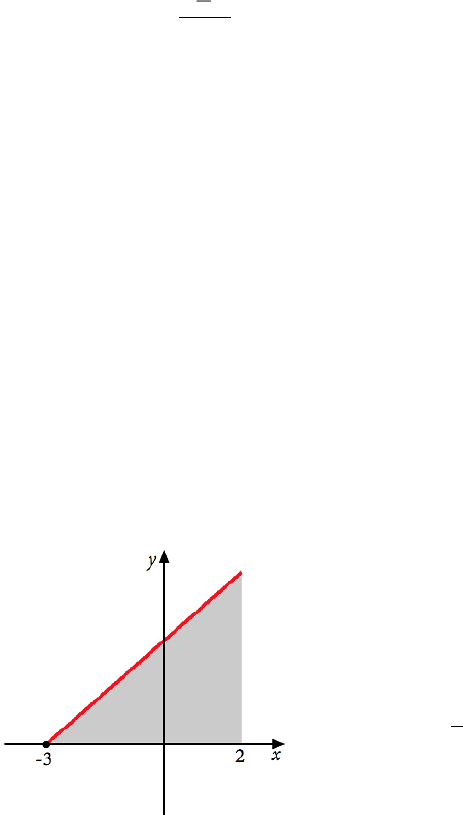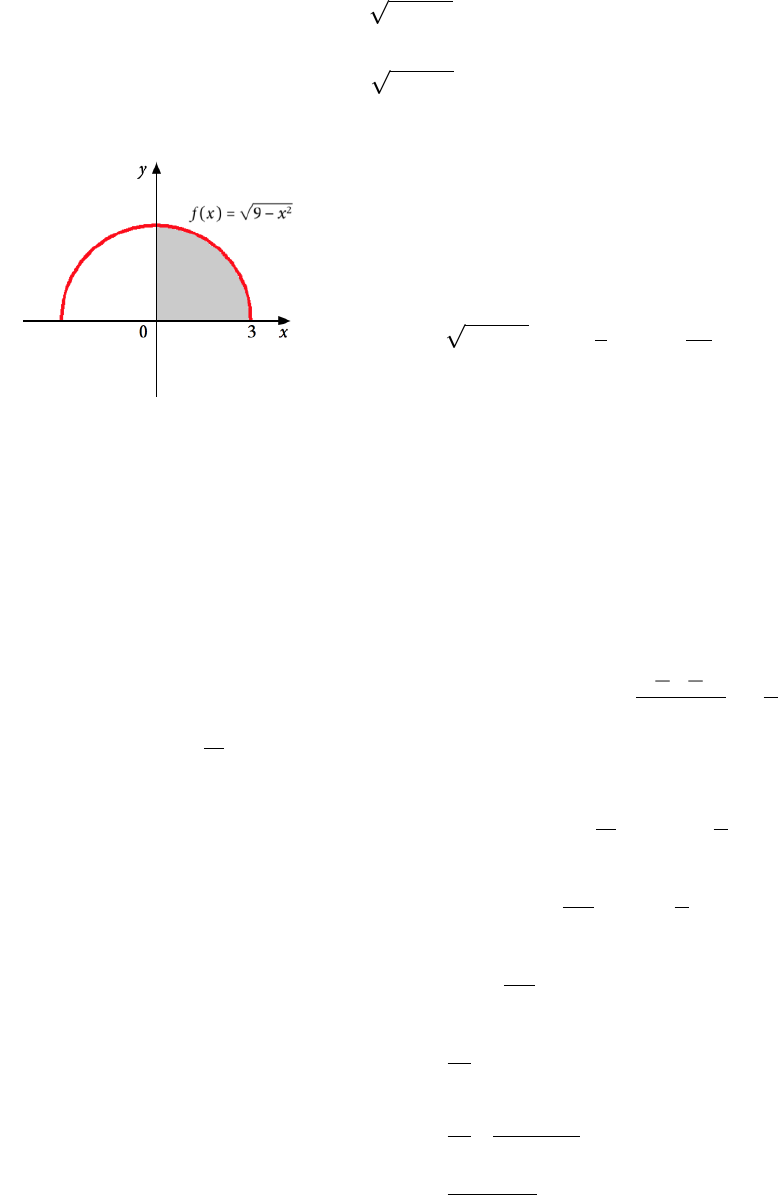Study Guides (400,000)
US (230,000)
NCSU (600)
MA (50)
MA 141 (40)
Final

# MA 141 Lecture 22: Definite IntegralExam

Department
Mathematics
Course Code
MA 141
Professor
Kevin Flores
Study Guide
Final

This preview shows page 1. to view the full 5 pages of the document.MA 141 Chapter 4
Section 4.2: Deﬁnite Integral
Now we will consider functions that may be above or below the x-axis. We will consider
rectangles above the x-axis to have positive signed area and rectangles below the x-axis to
have negative signed area. The Riemann sum will then calculate the net area.
Def: Let f(x)be deﬁned and continuous on the interval [a, b]. For each partition a=x0<
x1< x2< . . . < xn1< xn=bof the interval [a, b]into nequal parts, the length of each
subinterval is x=ba
nand each xi=a+ix,i= 1,2, . . . , n. The deﬁnite integral of
fon [a, b]is denoted and deﬁned by
Zb
a
f(x)dx = lim
n→∞
n
X
i=1
f(xi)x
provided this limit exists. If the limit exists, we call fintegrable on the interval [a, b].
Here, aand bare called the limits of integration. The term f(x)dx is a diﬀerential as in
Section 3.6.
Theorem: If f(x)is a continuous function on [a, b], then Zb
a
f(x)dx exists.
Ex: Evaluate the deﬁnite integral Z2
3
(2x+ 6)dx using geometry.
Let’s draw this function over the interval [3,2]:
= =
This region is a triangle with base b= 5
and height h=f(2) = 2(2) + 6 = 10.
Then the area of the shaded region is
A=1
2(5)(10) = 25
Since the deﬁnite integral Z2
3
(2x+ 6)dx =the area between the "curve" and the x-axis, we
know
Z2
3
(2x+ 6)dx = 25
1

Unlock to view full version

Only page 1 are available for preview. Some parts have been intentionally blurred.Ex: Evaluate the deﬁnite integral Z3
0
9x2dx using geometry.
Let’s graph again - what shape does 9x2have? This is the upper half of a circle of
radius 3, centered at the origin.
The full circle would have area π·32= 9π.
Since we are only concerned with the
quarter of the circle on the right, the area
is
Z3
0
9x2dx =1
4·9π=9π
4.
Ex: Evaluate the Z2
3
(2x+ 6)dx using the limit deﬁnition of the deﬁnite integral.
In the previous example, we showed this deﬁnite integral to be equal to 25 using geometry.
Let’s verify using the limit deﬁnition of deﬁnite integral.
Z2
3
(2x+ 6)dx = lim
n→∞
n
X
i=1
f(xi)x= lim
n→∞
n
X
i=1
(2xi+ 6)x
We need to ﬁnd xiand x. Since the interval is [3,2],x=2(3)
n=5
n. Recall that
xi=a+ix=3 + 5i
n.
lim
n→∞
n
X
i=1
(2xi+ 6)x= lim
n→∞
n
X
i=1 23 + 5i
n+ 6·5
n
= lim
n→∞
n
X
i=1 6 + 10i
n+ 6·5
n
= lim
n→∞
n
X
i=1 50i
n2
= lim
n→∞
50
n2
n
X
i=1
i
= lim
n→∞
50
n2·n(n+ 1)
2
= lim
n→∞
50n+ 50
2n
= 25